# Array Formulas

This chapter helps you understand array formulas in Excel. Single cell array formulas perform multiple calculations in one cell.

### Without Array Formula

Without using an array formula, we would execute the following steps to find the greatest progress.

1. First, we would calculate the progress of each student.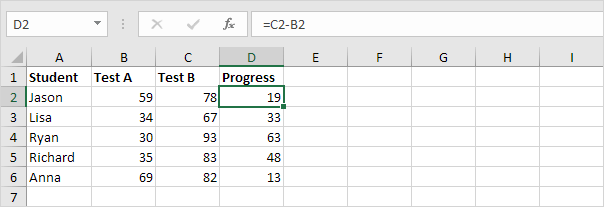2. Next, we would use the MAX function to find the greatest progress.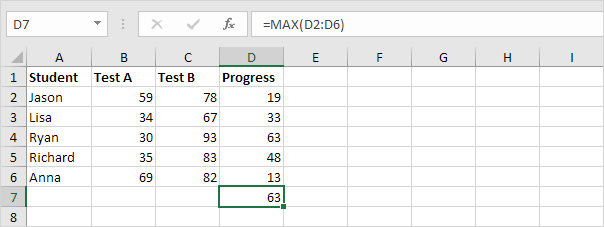### With Array Formula

We don't need to store the range in column D. Excel can store this range in its memory. A range stored in Excel's memory is called an array constant.

1. We already know that we can find the progress of the first student by using the formula below.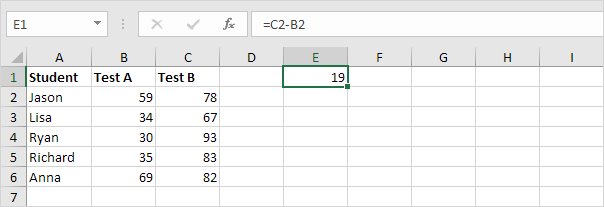2. To find the greatest progress (don't be overwhelmed), we add the MAX function, replace C2 with C2:C6 and B2 with B2:B6.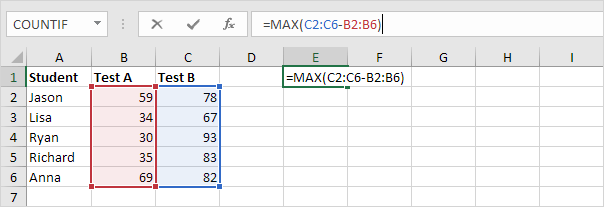3. Finish by pressing CTRL + SHIFT + ENTER.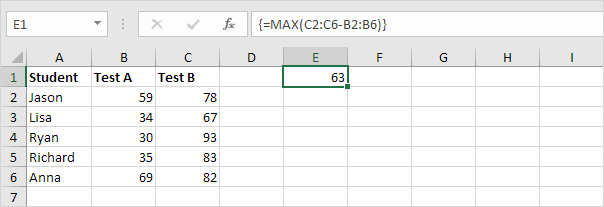Note: the formula bar indicates that this is an array formula by enclosing it in curly braces {}. Do not type these yourself. They will disappear when you edit the formula.

Explanation: the range (array constant) is stored in Excel's memory, not in a range. The array constant looks as follows:

{19;33;63;48;13}

This array constant is used as an argument for the MAX function, giving a result of 63.

4. If you're using Excel 365 or Excel 2021, finish by simply pressing Enter. Bye bye curly braces.### F9 Key

When working with array formulas, you can have a look at these array constants yourself.

1. Select C2:C6-B2:B6 in the formula.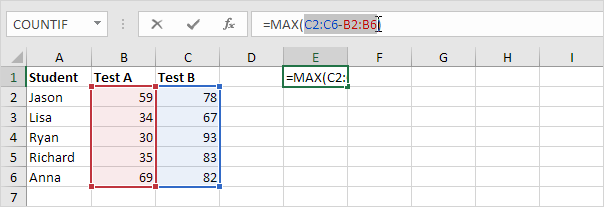2. Press F9.That looks good. Elements in a vertical array constant are separated by semicolons. Elements in a horizontal array constant are separated by commas.

Go to Next Chapter: Sort